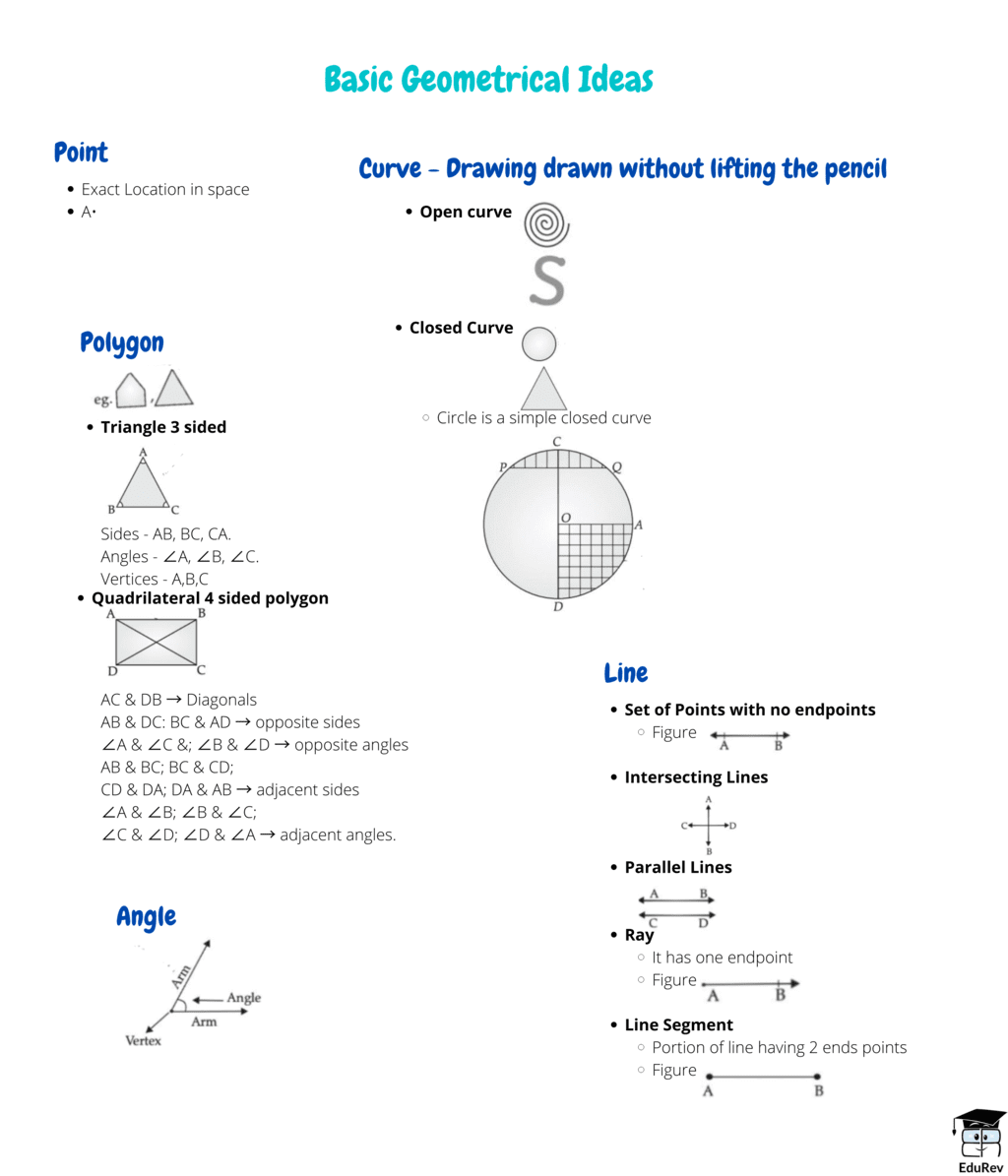Mindmap: Basic Geometrical Ideas

# Mindmap: Basic Geometrical Ideas Notes | Study Mathematics (Maths) Class 6 - Class 6

## Document Description: Mindmap: Basic Geometrical Ideas for Class 6 2022 is part of Mathematics (Maths) Class 6 preparation. The notes and questions for Mindmap: Basic Geometrical Ideas have been prepared according to the Class 6 exam syllabus. Information about Mindmap: Basic Geometrical Ideas covers topics like and Mindmap: Basic Geometrical Ideas Example, for Class 6 2022 Exam. Find important definitions, questions, notes, meanings, examples, exercises and tests below for Mindmap: Basic Geometrical Ideas.

Introduction of Mindmap: Basic Geometrical Ideas in English is available as part of our Mathematics (Maths) Class 6 for Class 6 & Mindmap: Basic Geometrical Ideas in Hindi for Mathematics (Maths) Class 6 course. Download more important topics related with notes, lectures and mock test series for Class 6 Exam by signing up for free. Class 6: Mindmap: Basic Geometrical Ideas Notes | Study Mathematics (Maths) Class 6 - Class 6
 1 Crore+ students have signed up on EduRev. Have you?The document Mindmap: Basic Geometrical Ideas Notes | Study Mathematics (Maths) Class 6 - Class 6 is a part of the Class 6 Course Mathematics (Maths) Class 6.
All you need of Class 6 at this link: Class 6

## Mathematics (Maths) Class 6

167 videos|275 docs|43 tests
 Use Code STAYHOME200 and get INR 200 additional OFF

## Mathematics (Maths) Class 6

167 videos|275 docs|43 tests

Track your progress, build streaks, highlight & save important lessons and more!

,

,

,

,

,

,

,

,

,

,

,

,

,

,

,

,

,

,

,

,

,

;